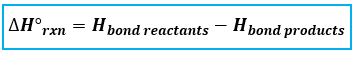# Problem: 2 H2S(g) + 3 O2(g) --&gt; 2 SO2(g) + 2 H2O(g)Calculate the ∆Hrxn in kJ.Average bond energies:H—S 347 kJ/molO = O 495 kJ/molO—H 463 kJ/mol

###### FREE Expert Solution
95% (178 ratings)
###### FREE Expert Solution

To calculate the ΔH°rxn using bond energies, we’re going to use the following equation:Let’s first figure out what kind of bonds and how many moles are present in each reactants and products because the given bond energies are per mole.

Balanced Reaction:  2 H2S(g) + 3 O2(g)  2 SO2(g) + 2 H2O(g)

*always make sure that the given reaction is balanced

Draw the structure of each compound.

Reactants:

H2S:

95% (178 ratings)###### Problem Details

2 H2S(g) + 3 O2(g) --> 2 SO2(g) + 2 H2O(g)

Calculate the ∆Hrxn in kJ.

Average bond energies:

H—S 347 kJ/mol

O = O 495 kJ/mol

O—H 463 kJ/mol

What scientific concept do you need to know in order to solve this problem?

Our tutors have indicated that to solve this problem you will need to apply the Bond Energy concept. You can view video lessons to learn Bond Energy. Or if you need more Bond Energy practice, you can also practice Bond Energy practice problems.

What is the difficulty of this problem?

Our tutors rated the difficulty of2 H2S(g) + 3 O2(g) --> 2 SO2(g) + 2 H2O(g)Calculate the ∆...as high difficulty.

How long does this problem take to solve?

Our expert Chemistry tutor, Dasha took 6 minutes and 43 seconds to solve this problem. You can follow their steps in the video explanation above.

What professor is this problem relevant for?

Based on our data, we think this problem is relevant for Professor Bell's class at PITT.